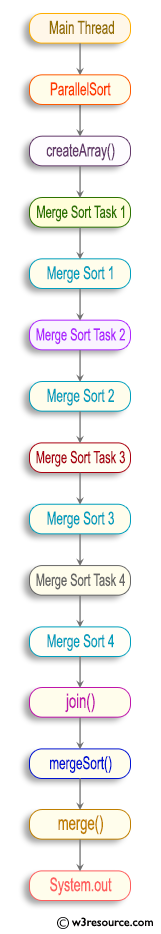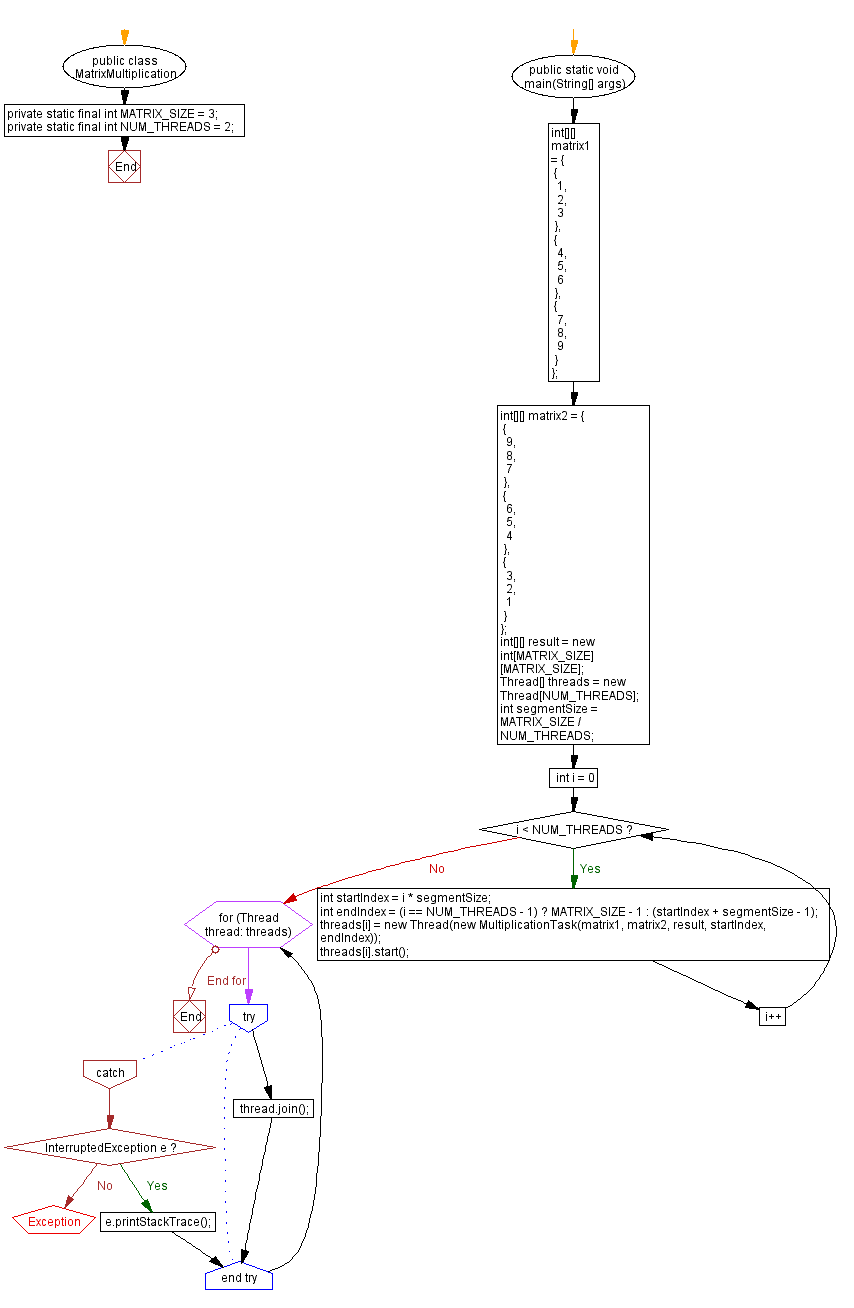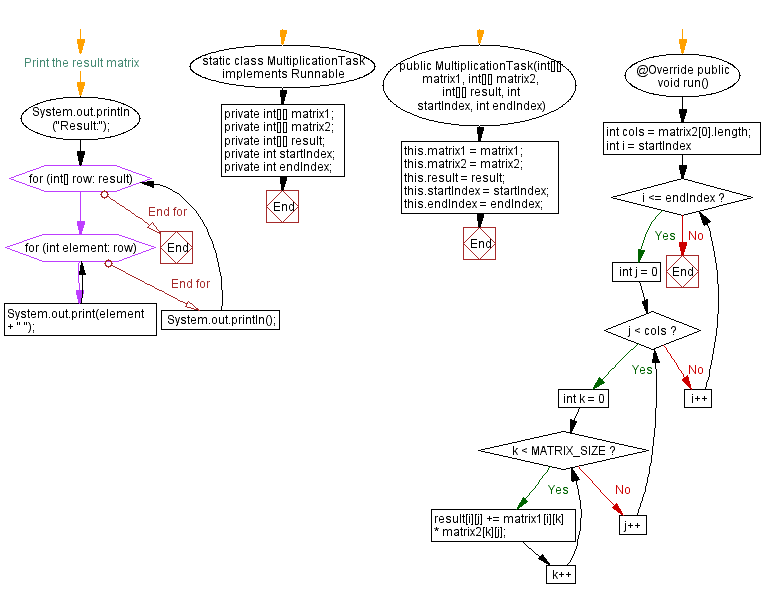﻿ Multithreaded Java Program: Matrix Multiplication

## Java Thread: Exercise-4 with Solution

Write a Java program that performs matrix multiplication using multiple threads.

Sample Solution:

Java Code:

``````public class MatrixMultiplication {
private static final int MATRIX_SIZE = 3;
private static final int NUM_THREADS = 2;

public static void main(String[] args) {
int[][] matrix1 = {
{
1,
2,
3
},
{
4,
5,
6
},
{
7,
8,
9
}
};

int[][] matrix2 = {
{
9,
8,
7
},
{
6,
5,
4
},
{
3,
2,
1
}
};

int[][] result = new int[MATRIX_SIZE][MATRIX_SIZE];

int segmentSize = MATRIX_SIZE / NUM_THREADS;

for (int i = 0; i < NUM_THREADS; i++) {
int startIndex = i * segmentSize;
int endIndex = (i == NUM_THREADS - 1) ? MATRIX_SIZE - 1 : (startIndex + segmentSize - 1);
}

try {
} catch (InterruptedException e) {
e.printStackTrace();
}
}

// Print the result matrix
System.out.println("Result:");
for (int[] row: result) {
for (int element: row) {
System.out.print(element + " ");
}
System.out.println();
}
}

static class MultiplicationTask implements Runnable {
private int[][] matrix1;
private int[][] matrix2;
private int[][] result;
private int startIndex;
private int endIndex;

public MultiplicationTask(int[][] matrix1, int[][] matrix2, int[][] result, int startIndex, int endIndex) {
this.matrix1 = matrix1;
this.matrix2 = matrix2;
this.result = result;
this.startIndex = startIndex;
this.endIndex = endIndex;
}

@Override
public void run() {
int cols = matrix2.length;

for (int i = startIndex; i <= endIndex; i++) {
for (int j = 0; j < cols; j++) {
for (int k = 0; k < MATRIX_SIZE; k++) {
result[i][j] += matrix1[i][k] * matrix2[k][j];
}
}
}
}
}
}
```
```

Sample Output:

``` Result:
78 96 114
186 240 294
294 384 474
```Explanation:

In the above exercise,

• The Matrix_Multiplication class performs matrix multiplication operation.
• The program begins by defining the size of the matrix (MATRIX_SIZE) and the number of threads to use (NUM_THREADS).
• Two input matrices, matrix1 and matrix2, are declared and initialized with their respective values.
• An empty result matrix is created to store the matrix multiplication result.
• An array of threads, threads, is created to handle matrix multiplication. The number of rows in the matrixes is divided into segments based on the number of threads. Each thread multiplies a specific segment of the matrix.
• In a loop, the start and end indexes are calculated for each thread based on the segment size. The threads are created and started, with each thread executing the Multiplication_Task class.
• Another loop waits for all threads to complete their execution using the join() method.
• The result matrix is printed by iterating through its rows and columns.
• The Multiplication_Task class represents the matrix multiplication task performed by each thread. It takes input matrices, the result matrix, start index, and end index as input parameters. In the run() method, each thread performs matrix multiplication for the rows in the result matrix. It uses nested loops to iterate through the rows and columns of the matrices and calculates the corresponding element of the result matrix.

Flowchart:Java Code Editor:

Improve this sample solution and post your code through Disqus

What is the difficulty level of this exercise?

Test your Programming skills with w3resource's quiz.

﻿

## Java: Tips of the Day

Converts a string from camelcase:

```public static String fromCamelCase(String input, String separator) {
return input
.replaceAll("([a-z\\d])([A-Z])", "\$1" + separator + "\$2")
.toLowerCase();
}
```

Ref: https://bit.ly/3u09A9E

We are closing our Disqus commenting system for some maintenanace issues. You may write to us at reach[at]yahoo[dot]com or visit us at Facebook